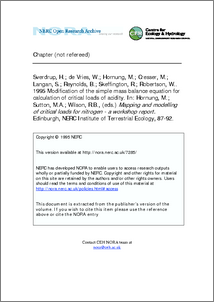nerc.ac.uk

# Modification of the simple mass balance equation for calculation of critical loads of acidity.

Sverdrup, H.; de Vries, W.; Hornung, M.; Cresser, M.; Langan, S.; Reynolds, B.; Skeffington, R.; Robertson, W.. 1995 Modification of the simple mass balance equation for calculation of critical loads of acidity. In: Hornung, M.; Sutton, M.A.; Wilson, R.B., (eds.) Mapping and modelling of critical loads for nitrogen - a workshop report. Edinburgh, NERC Institute of Terrestrial Ecology, 87-92.Preview
Text
N007285CP.pdf

## Abstract/Summary

Over the last few years, the simple mass balance equation for the calculation of critical loads of acidity has been gradually modified as the underlying critical load concepts have developed and as problems with particular forms of the equation have been identified, through application in particular countries. The first major update of the equation took place following a workshop held in Vienna, Austria (Hojesky et al. 1993). The workshop was held to discuss problems which had been identified when the then current form of the equation was applied in countries with high rainfall. The problems had largely arisen because of simplifications and assumptions incorporated into the early formulation of the equation. The equation was reformulated to overcome the problems identified at the workshop. However, further problems were identified when the reformulated equation was applied in the UK in situations with a combination of high rainfall, large marine inputs and widespread occurrence of organic soils. A small workshop was, therefore held in Grange-over-Sands, UK in late 1993 to dicuss the problems and to further re-evaluate the equation. The problems had arisen in the UK because of simplifications and assumptions made in the formulation concerning, in particular, cation leaching and uptake. As a result, a more rigorous treatment of these variables was incorporated into the equation. The reformulation of the equation, as derived at the September 1993 workshop is described below.

Item Type: Publication - Book Section CEH Programmes pre-2009 publications > Other _ Pre-2000 sections 1870393244 Scanned legacy/working document Nitrogen, Deposition, Freshwater, Sulphur, Equation HydrologyChemistryAtmospheric Sciences 29 May 2009 07:48 +0 (UTC) http://nora.nerc.ac.uk/id/eprint/7285View Item